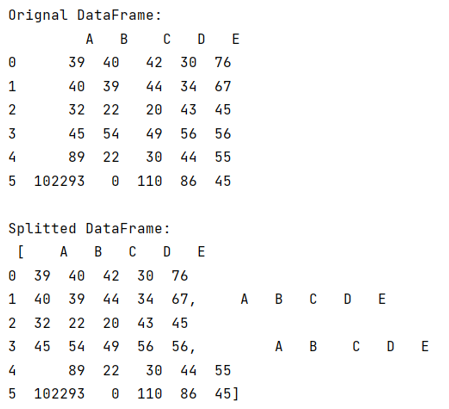# Split a large pandas DataFrame

Given a Pandas DataFrame, we have to split it.
Submitted by Pranit Sharma, on June 23, 2022

Pandas is a special tool that allows us to perform complex manipulations of data effectively and efficiently. Inside pandas, we mostly deal with a dataset in the form of DataFrame. DataFrames are 2-dimensional data structures in pandas. DataFrames consist of rows, columns, and the data.

## Splitting a DataFrame

Splitting a DataFrame means breaking a DataFrame into multiple parts for a better understanding of the data and effective data analysis.

For splitting a DataFrame, we use the NumPy package, NumPy module has a method called array_split.

To work with pandas, we need to import pandas package first, below is the syntax:

```import pandas as pd
```

Let us understand with the help of an example,

## Python code to split a large pandas DataFrame

```# Importing pandas package
import pandas as pd

# Importing random package
import random

# Importing numpy package
import numpy as np

# Create a DataFrame
df = pd.DataFrame({
'A':[39,40,32,45,89,102293],
'B':[40,39,22,54,22,0],
'C':[42,44,20,49,30,110],
'D':[30,34,43,56,44,86],
'E':[76,67,45,56,55,45]
})

# Display original DataFrame
print("Orignal DataFrame:\n",df,"\n")

# Splitting DataFrame
result = np.array_split(df, 3)

# Display result
print("Splitted DataFrame:\n",result,"\n")
```

Output:What's New

Top Interview Coding Problems/Challenges!

Languages: » C » C++ » C++ STL » Java » Data Structure » C#.Net » Android » Kotlin » SQL
Web Technologies: » PHP » Python » JavaScript » CSS » Ajax » Node.js » Web programming/HTML
Solved programs: » C » C++ » DS » Java » C#
Aptitude que. & ans.: » C » C++ » Java » DBMS
Interview que. & ans.: » C » Embedded C » Java » SEO » HR
CS Subjects: » CS Basics » O.S. » Networks » DBMS » Embedded Systems » Cloud Computing
» Machine learning » CS Organizations » Linux » DOS
More: » Articles » Puzzles » News/Updates